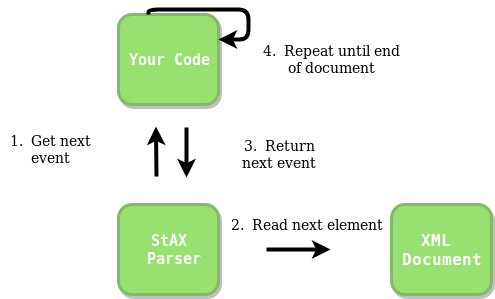# StAX XML Parser in Java

• Last Updated : 29 Dec, 2021

This article focuses on how one can parse a XML file in Java.
XML : XML stands for eXtensible Markup Language. It was designed to store and transport data. It was designed to be both human- and machine-readable. That’s why, the design goals of XML emphasize simplicity, generality, and usability across the Internet.

Why StAX instead of SAX ?

• SAX: The SAX is a push model API which means that it is the API which calls your handler, not your handler that calls the API . The SAX parser thus “pushes” events into your handler. With this push model of API you have no control over how and when the parser iterates over the file. Once you start the parser, it iterates all the way until the end, calling your handler for each and every XML event in the input XML document.
`SAX Parser --> Handler`
• StAX :  The StAX pull model means that it is your “handler” class that calls the parser API , not the other way around. Thus your handler class controls when the parser is to move on to the next event in the input. In other words, your handler “pulls” the XML events out of the parser. Additionally, you can stop the parsing at any point. The StAX parser is generally used instead of a file reader , when the input or database is given in the form of offline or online xml file. The pull model of is summarized like this:
`Handler --> StAX Parser`
• Also StAX parser can read and write in the XML documents while SAX can only read. SAX provides the schema validation i.e. if the tags are nested correctly or XML is correctly written , but StAX provides no such method of schema validation.

Implementation

Idea of How StAX parser works :Input File : This is sample input file made by the author as an example to show how StAX parser is used . Save it as data.xml and run the code . XML database files usually are large and contains many tags nested within each other .

## Java

 `// Java Code to implement StAX parser``import` `java.io.File;``import` `java.io.FileNotFoundException;``import` `java.io.FileReader;``import` `java.util.Iterator;``import` `javax.xml.namespace.QName;``import` `javax.xml.stream.XMLEventReader;``import` `javax.xml.stream.XMLInputFactory;``import` `javax.xml.stream.XMLStreamException;``import` `javax.xml.stream.events.*;` `public` `class` `Main``{` `    ``private` `static` `boolean` `bcompany,btitle,bname,bemail,bphone;` `    ``public` `static` `void` `main(String[] args) ``throws` `FileNotFoundException,``                                                ``XMLStreamException``    ``{``        ``// Create a File object with appropriate xml file name``        ``File file = ``new` `File(``"data.xml"``);` `        ``// Function for accessing the data``        ``parser(file);``    ``}` `    ``public` `static` `void` `parser(File file) ``throws` `FileNotFoundException,``                                                ``XMLStreamException``    ``{``        ``// Variables to make sure whether a element``        ``// in the xml is being accessed or not``        ``// if false that means elements is``        ``// not been used currently , if true the element or the``        ``// tag is being used currently``        ``bcompany = btitle = bname = bemail = bphone = ``false``;` `        ``// Instance of the class which helps on reading tags``        ``XMLInputFactory factory = XMLInputFactory.newInstance();` `        ``// Initializing the handler to access the tags in the XML file``        ``XMLEventReader eventReader =``                ``factory.createXMLEventReader(``new` `FileReader(file));` `        ``// Checking the availability of the next tag``        ``while` `(eventReader.hasNext())``        ``{``            ``// Event is actually the tag . It is of 3 types``            ``// = StartEvent``            ``// = EndEvent``            ``// data between the StartEvent and the EndEvent``            ``// which is Characters Event``            ``XMLEvent event = eventReader.nextEvent();` `            ``// This will trigger when the tag is of type <...>``            ``if` `(event.isStartElement())``            ``{``                ``StartElement element = (StartElement)event;` `                ``// Iterator for accessing the metadeta related``                ``// the tag started.``                ``// Here, it would name of the company``                ``Iterator iterator = element.getAttributes();``                ``while` `(iterator.hasNext())``                ``{``                    ``Attribute attribute = iterator.next();``                    ``QName name = attribute.getName();``                    ``String value = attribute.getValue();``                    ``System.out.println(name+``" = "` `+ value);``                ``}` `                ``// Checking which tag needs to be opened for reading.``                ``// If the tag matches then the boolean of that tag``                ``// is set to be true.``                ``if` `(element.getName().toString().equalsIgnoreCase(``"company"``))``                ``{``                    ``bcompany = ``true``;``                ``}``                ``if` `(element.getName().toString().equalsIgnoreCase(``"title"``))``                ``{``                    ``btitle = ``true``;``                ``}``                ``if` `(element.getName().toString().equalsIgnoreCase(``"name"``))``                ``{``                    ``bname = ``true``;``                ``}``                ``if` `(element.getName().toString().equalsIgnoreCase(``"email"``))``                ``{``                    ``bemail = ``true``;``                ``}``                ``if` `(element.getName().toString().equalsIgnoreCase(``"phone"``))``                ``{``                    ``bphone = ``true``;``                ``}``            ``}` `            ``// This will be triggered when the tag is of type ``            ``if` `(event.isEndElement())``            ``{``                ``EndElement element = (EndElement) event;` `                ``// Checking which tag needs to be closed after reading.``                ``// If the tag matches then the boolean of that tag is``                ``// set to be false.``                ``if` `(element.getName().toString().equalsIgnoreCase(``"company"``))``                ``{``                    ``bcompany = ``false``;``                ``}``                ``if` `(element.getName().toString().equalsIgnoreCase(``"title"``))``                ``{``                    ``btitle = ``false``;``                ``}``                ``if` `(element.getName().toString().equalsIgnoreCase(``"name"``))``                ``{``                    ``bname = ``false``;``                ``}``                ``if` `(element.getName().toString().equalsIgnoreCase(``"email"``))``                ``{``                    ``bemail = ``false``;``                ``}``                ``if` `(element.getName().toString().equalsIgnoreCase(``"phone"``))``                ``{``                    ``bphone = ``false``;``                ``}``            ``}` `            ``// Triggered when there is data after the tag which is``            ``// currently opened.``            ``if` `(event.isCharacters())``            ``{``                ``// Depending upon the tag opened the data is retrieved .``                ``Characters element = (Characters) event;``                ``if` `(bcompany)``                ``{``                    ``System.out.println(element.getData());``                ``}``                ``if` `(btitle)``                ``{``                    ``System.out.println(element.getData());``                ``}``                ``if` `(bname)``                ``{``                    ``System.out.println(element.getData());``                ``}``                ``if` `(bemail)``                ``{``                    ``System.out.println(element.getData());``                ``}``                ``if` `(bphone)``                ``{``                    ``System.out.println(element.getData());``                ``}``            ``}``        ``}``    ``}``}`

Output :

```name = geeksforgeeks.org
Kunal Sharma
Student
kunal@example.com
(202) 456-1414```

How does StAX work in the above Code ?

After creating the eventReader in the above code with the help of factory pattern to create a XML file reader, it basically starts by reading the <…> tag . As soon as <…> tag comes, a boolean variable is set to true indicating that the tag has been opened. This tag matching is done by identifying whether it is a start tag or end tag. Since <…> tag indicates the starting, therefore it is matched by StartElement. Next comes the data reading part. In the next step, it reads the character/data by matching the element by isCharacters, this is done only if the starting tag that we require is opened or its boolean variable is set true. After this comes closing of element indicated by </…> tag. As soon it encounters </..> it checks which of the elements was opened or set to true and it sets that element boolean to false or closes it.

Basically each event is first opening the tag, reading its data and then closing it.

This article is contributed by Kunal Sharma. If you like GeeksforGeeks and would like to contribute, you can also write an article using write.geeksforgeeks.org or mail your article to review-team@geeksforgeeks.org. See your article appearing on the GeeksforGeeks main page and help other Geeks.